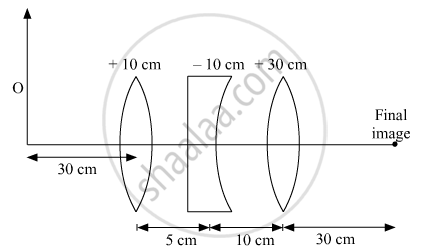# Three Lenses of Focal Length +10 Cm, —10 Cm and +30 Cm Are Arranged Coaxially as in the Figure Given Below. Find the Position of the Final Image Formed by the Combination. - Physics

Numerical

Three lenses of focal length +10 cm, —10 cm and +30 cm are arranged coaxially as in the figure given below. Find the position of the final image formed by the combination.#### Solution1/v - 1/u = 1/f

u = -30 cm

f = +10 cm

1/nu = 1/f + 1/u

1/nu_1 = 1/10 - 1/30

⇒ 1/nu_1 = (3 - 1)/30 = 2/30

nu_1 = 15 cm

u = +10 cm

f = -10 cm

1/nu_2 = 1/f + 1/u = 1/10 - 1/10

nu_2  = ∞

For third lens object is at infinity hence image is formed at focus final image at a distance of 30 cm.

Is there an error in this question or solution?
2018-2019 (March) 55/1/3

Share